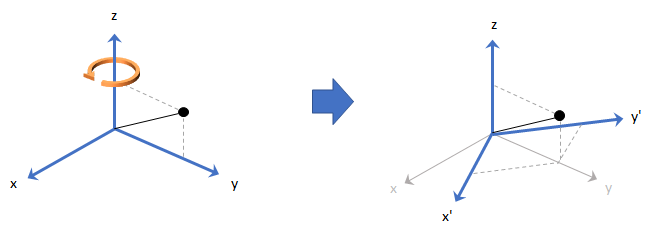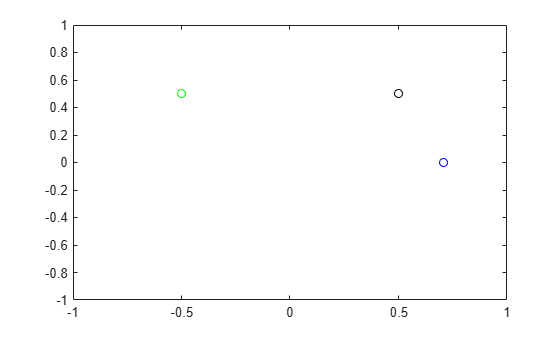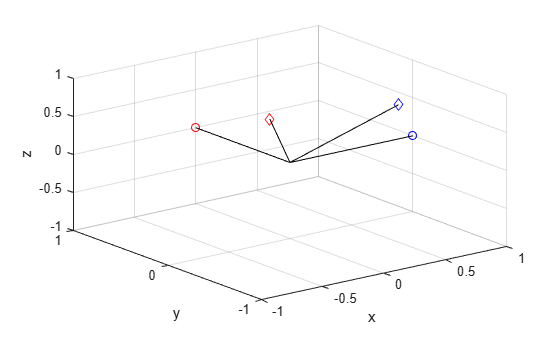rotateframe

Quaternion frame rotation

Description

example

rotationResult = rotateframe(quat,cartesianPoints) rotates the frame of reference for the Cartesian points using the quaternion, quat. The elements of the quaternion are normalized before use in the rotation.Examples

collapse all

Define a point in three dimensions. The coordinates of a point are always specified in the order x, y, and z. For convenient visualization, define the point on the x-y plane.

x = 0.5;
y = 0.5;
z = 0;
plot(x,y,'ko')
hold on
axis([-1 1 -1 1])Create a quaternion vector specifying two separate rotations, one to rotate the frame 45 degrees and another to rotate the point -90 degrees about the z-axis. Use rotateframe to perform the rotations.

quat = quaternion([0,0,pi/4; ...
0,0,-pi/2],'euler','XYZ','frame');

rereferencedPoint = rotateframe(quat,[x,y,z])
rereferencedPoint = 2×3

0.7071   -0.0000         0
-0.5000    0.5000         0

Plot the rereferenced points.

plot(rereferencedPoint(1,1),rereferencedPoint(1,2),'bo')
plot(rereferencedPoint(2,1),rereferencedPoint(2,2),'go')Define two points in three-dimensional space. Define a quaternion to rereference the points by first rotating the reference frame about the z-axis 30 degrees and then about the new y-axis 45 degrees.

a = [1,0,0];
b = [0,1,0];
quat = quaternion([30,45,0],'eulerd','ZYX','point');

Use rotateframe to reference both points using the quaternion rotation operator. Display the result.

rP = rotateframe(quat,[a;b])
rP = 2×3

0.6124   -0.3536    0.7071
0.5000    0.8660   -0.0000

Visualize the original orientation and the rotated orientation of the points. Draw lines from the origin to each of the points for visualization purposes.

plot3(a(1),a(2),a(3),'bo');

hold on
grid on
axis([-1 1 -1 1 -1 1])
xlabel('x')
ylabel('y')
zlabel('z')

plot3(b(1),b(2),b(3),'ro');
plot3(rP(1,1),rP(1,2),rP(1,3),'bd')
plot3(rP(2,1),rP(2,2),rP(2,3),'rd')

plot3([0;rP(1,1)],[0;rP(1,2)],[0;rP(1,3)],'k')
plot3([0;rP(2,1)],[0;rP(2,2)],[0;rP(2,3)],'k')
plot3([0;a(1)],[0;a(2)],[0;a(3)],'k')
plot3([0;b(1)],[0;b(2)],[0;b(3)],'k')Input Arguments

collapse all

Quaternion that defines rotation, specified as a scalar quaternion or vector of quaternions.

Data Types: quaternion

Three-dimensional Cartesian points, specified as a 1-by-3 vector or N-by-3 matrix.

Data Types: single | double

Output Arguments

collapse all

Cartesian points defined in reference to rotated reference frame, returned as a vector or matrix the same size as cartesianPoints.

The data type of the re-referenced Cartesian points is the same as the underlying data type of quat.

Data Types: single | double

Algorithms

Quaternion frame rotation re-references a point specified in R3 by rotating the original frame of reference according to a specified quaternion:

${L}_{q}\left(u\right)={q}^{*}uq$

where q is the quaternion, * represents conjugation, and u is the point to rotate, specified as a quaternion.

For convenience, the rotateframe function takes a point in R3 and returns a point in R3. Given a function call with some arbitrary quaternion, q = a + bi + cj + dk, and arbitrary coordinate, [x,y,z],

point = [x,y,z];
rereferencedPoint = rotateframe(q,point)
the rotateframe function performs the following operations:

1. Converts point [x,y,z] to a quaternion:

${u}_{q}=0+xi+yj+zk$

2. Normalizes the quaternion, q:

${q}_{n}=\frac{q}{\sqrt{{a}^{2}+{b}^{2}+{c}^{2}+{d}^{2}}}$

3. Applies the rotation:

${v}_{q}={q}^{*}{u}_{q}q$

4. Converts the quaternion output, vq, back to R3